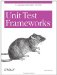# 4.3 Single Condition Tests

A useful rule of thumb is that a test method should only contain a single test assertion. The idea is that a test method should only test one behavior; if there is more than one assert condition, multiple things are being tested . When there is more than one condition to test, then a test fixture should be set up, and each condition placed in a separate test method.

The xUnits tend to enforce this rule when handling test assertion failures. A test method returns as soon as a failure occurs, skipping any additional code. Running the rest of the test is unnecessary, since the result (failure) is known.

Practically speaking, test methods containing several assertions are not always a terrible thing. Tests may have conditions that can only be combined into one expression with unnecessary complication of the code. The testGetBooks( ) method in the previous section verifies that the Library contains two Book s, which is most clearly expressed as two separate asserts, although they could be combined into one compound condition. A single behavior can have several side effects that you should check with separate assertions. So, it's not a problem when a test method contains several asserts, as long as the test method is only testing a single behavior.

However, a test method with many asserts is a clear indicator that a single test is doing too much. Example 4-6 shows a test method with this problem.

##### Example 4-6. Poorly written unit test that tests multiple behaviors
` LibraryTest.java    public void  testLookupBooksByAuthor( )  {       // Add two books by same author       Book book3 = new Book( "Cosmos", "Carl Sagan" );       Book book4 = new Book( "Contact", "Carl Sagan" );       library.addBook( book3 );       library.addBook( book4 );       // Look up books by title and author       Book book = library.getBook( "Cosmos", "Carl Sagan" );       BookTest.assertEquals( book3, book );       book = library.getBook( "Contact", "Carl Sagan" );       BookTest.assertEquals( book4, book );       // Look up both books by author       Vector books = library.getBooks( "Carl Sagan" );       assertEquals( "two books not found", 2, books.size( ) );       book = (Book)books.elementAt(0);       BookTest.assertEquals( book3, book );       book = (Book)books.elementAt(1);       BookTest.assertEquals( book4, book );    } `

How is this test flawed? Let us count the ways. It tests two separate behaviors: getting a Book by author and title and getting multiple Book s by the same author. Looking up two books by two different methods means there are several results to test; thus, there are many assertsfive in all. Although it is sensible to check the results of all the operations, there are redundant tests, such as the two tests of the getBook() method. To get the test to pass, numerous changes must be made immediately to both Book and Library . The complexity of the changes increases the chance that a coding mistake will be made. When one assert in the sequence fails, the rest will be skipped , leaving it uncertain whether those asserts would succeed. So, if the Book lookup by title and author fails, it has to be fixed before the test that gets multiple Book s is run. In other words, the tests are coupled so that failure of one may affect the success of the others.

When the number of asserts in a test method is excessive, change it into a test fixture with multiple test methods, each testing one behavior. In Example 4-7, refactoring the test method makes it apparent that the two lookup methods are distinct behaviors and should be tested separately.

##### Example 4-7. The previous test method refactored into separate test methods
` LibraryTest.java    public void setUp( ) {  book3 = new Book( "Cosmos", "Carl Sagan" );   book4 = new Book( "Contact", "Carl Sagan" );   library.addBook( book3 );   library.addBook( book4 );  }    public void  testGetBookByTitleAndAuthor( )  {       Book book = library.getBook( "Cosmos", "Carl Sagan" );       BookTest.assertEquals( book3, book );    }    public void  testGetBooksByAuthor( )  {       Vector books = library.getBooks( "Carl Sagan" );       assertEquals( "two books not found", 2, books.size( ) );       Book book = (Book)books.elementAt(0);       BookTest.assertEquals( book3, book );       book = (Book)books.elementAt(1);       BookTest.assertEquals( book4, book );    } `

Example 4-7 shows LibraryTest with the two separate test methods, one for each behavior. The code to add the two test Book s is placed in the setUp( ) method. The tests are isolated and the code is simplified.Unit Test Frameworks
ISBN: 0596006896
EAN: 2147483647
Year: 2006
Pages: 146
Authors: Paul Hamill